## Sunday, September 16, 2012

### What's wrong with Group Theory

I think I figured out what's wrong with group theory. Oh, there's nothing wrong with it; it's all intricately worked out to the ultimate degree. But there's something wrong with the way it's done. Mathematicians are all proud of themselves when they can reduce something to its barest essentials, and that's what they do to group theory.

Group theory has its origins in the theory of permutations. If you have four arbitrary elements, you can re-arrange them in 24 different ways. That's not hard to see. But the interesting thing is that among those re-arrangments, there is a certain structure. For example, the simplest re-arrangment on four elements is perhaps to move each one over one place until you get to the end, and take that one an bring it to the front. If you arranged the elements in a circle, you would just be rotating the whole array one notch. If this is the only operation you permit yourself, then there will be only four possilbe arrangements of your group elements: ABCD, DABC, CDAB, and BCDA.

Another very simple group operation would be to flip the first two elements with each other, leaving the other two where they were. If this is the only operation you allow yourself, then there will be only two possible arrangmenets: ABCD and BACD.

But what if you allow both of those operations? What are the possible outcomes? In particular, using just the flip and the rotation, can you then generate all twenty-four possible permutations of the four letters? That is the kind of question that group theory deals with.

By the way, it's easy to see that the order of operations makes a difference. If you flip first and then rotate, you get DBAC. If you rotate first and then flip, you get ADBC. So the algebra of the group is not, in general, "commutative". Maybe you can see why the Rubik's Cube is subject to analysis by group theory. My own interest is in group theory as applied to algebra of equations: this is the topic of Galois Theory.

Where mathematicians go wrong is they first develop group theory in its most abstract form, developing some very "powerful" theorems along the way. And then they apply those theorems to Galois theory, and prove amazing things about the solvability of equations.

So what's wrong with that? Remember I said they start by developing group theory "in its most abstract form". And one of the manifestations of that abstractness is that they say that the "algebra" of permutation theory doesn't depend on those arbitrary elements which you are shuffling about. (I put "algebra" in quotes to distinguish it from the ordinary meaning of algebra as applied to numbers.) We can develop the whole theory just by looking at the relation between our operations, and ignoring the "things" that they are operating on.

For example, look at our flips and rotations, which we can call F and R. If we flip twice in a row, we get back where we started. So we can say F^2=1: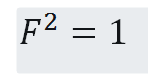Similarly, if we rotate four times, we get back to where we started. So we can say R^4=1: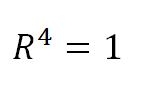We need one more fact before we can proceed...something to connect F and R. By fiddling around, we can observe that if we rotate, flip and rotate again, it's the same as flipping, rotating backwards (the same as rotating forward three times) and then flipping. In other words: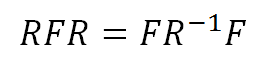From these three relationships we can develop the complete algebra of the group, without any further reference to shuffling about the letters A,B,C,D. For example, using only these relationships we can demonstrate the existence of an element O = RF such that O^3 = 1: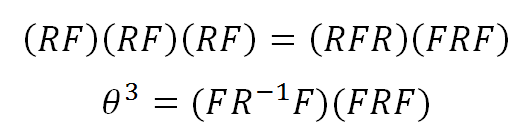To reduce this equation, we can think pictorially and remember that flipping something twice in a row just brings us back where we started: or we can just use the formal relationship F^2=1 which we took as one of the defining properties of our group algebra. Either way: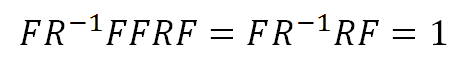So purely by formal manipulations, we have shown that there is a group element which returns our original configuration when applied three times in a row. If we look at our pictorial representation, we can see why. If we take the letters ABCD, rotate and then flip the first two, we get the configuration ADBC: in other words, A stayed where it was, and the letters BCD rotated clockwise. Obviously, three rotations of the letters BCD will return us to ABCD. But somehow, it seems to be more in the spirit of Group Theory, as it is practised within the academic community, to prove this by formal manipulations of symbols.

Am I being quite fair in this? Aren't mathematicians eager to use pictures and graphic constructions whenever they can in order to make their results more understandable? You would normally think so, but I seem to have stumbled across a glaring exception which arises in the critical overlap between Group Theory and Galois Theory. It relates to something called a Quotient Group, which is normally given a very cryptic definition in formal group theory. The irony is that as it applies to Galois Theory, the whole concept of quotient groups makes total sense when it is defined in terms of the letters A,B,C and D which the group operates on: but this intuitive connection is needlessly ignored because the practitioners of Group Theory take enormous pride in their ability to define everything in terms of the F's and R's, so to speak, while ignoring the ABCD's which were the original motivation.

I'll come back to this when I return.